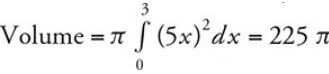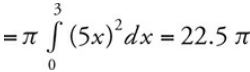# How to use a graphing calculator on AP calculus BC Exam

The use of a graphing calculator is permitted in Section I-Part B multiple?choice questions and in Section II-Part A free-response questions.

You are permitted to use the following 4 built-in capabilities of your graphing calculator to obtain an answer:

1. plotting the graph of a function

2. finding the zeros of a function

3. calculating numerically the derivative of a function

4. calculating numerically the value of a definite integral

For example, if you have to find the area of a region, you need to show a definite integral. You may then proceed to use the calculator to produce the numerical value of the definite integral without showing any supporting work. All other capabilities of your calculator can only be used to check your answer. For example, you may not use the built-in [Inflection] function of your calculator to find points of inflection. You must use calculus showing derivatives and indicating a change of concavity.

You may not use calculator syntax to substitute for calculus notations. For example, you may not write "Volume = ∫ (π(5x)∧ 2, x, 0,3) = 225 π", instead you need to write "".

When using a graphing calculator to solve a problem, you are required to write the setup that leads to the answer. For example, if you are finding the volume of a solid, you must write the definite integral and then use the calculator to compute the numerical value, e.g., Volume. Simply indicating the answer without writing the integral is considered an incomplete solution, for which you would receive minimal credit (possibly 1 point) instead of full credit for a complete solution.

Set your calculator to radian mode, and change to degree mode only if necessary.

If you are using a TI-89 graphing calculator, clear all previous entries for variables a through z before the AP Calculus BC exam.

You are permitted to store computer programs in your calculator and use them in the AP Calculus BC Exam. Your calculator memories will not be cleared.

Using the [Trace] function to find points on a graph may not produce the required accuracy. Most graphing calculators have other built-in functions that can produce more accurate results. For example, to find the xintercepts of a graph, use the [Zero] function, and to find the intersection point of two curves, use the [Intersection] function.

When decimal numbers are involved, do not round until the final answer. Unless otherwise stated, your final answer should be accurate to three places after the decimal point.

You may bring up to two calculators to the AP Calculus BC exam.

Replace old batteries with new ones and make sure that the calculator is functioning properly before the exam